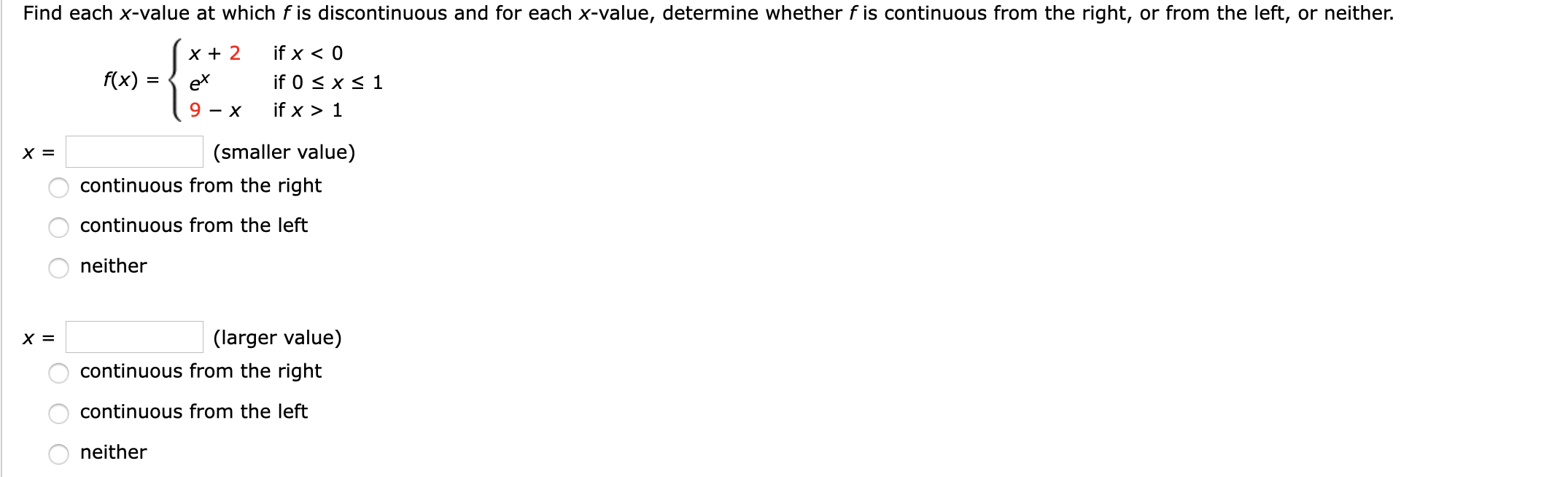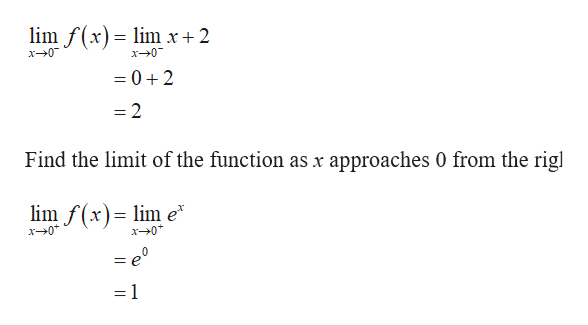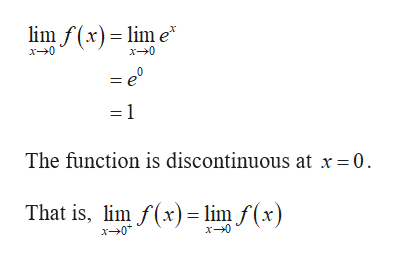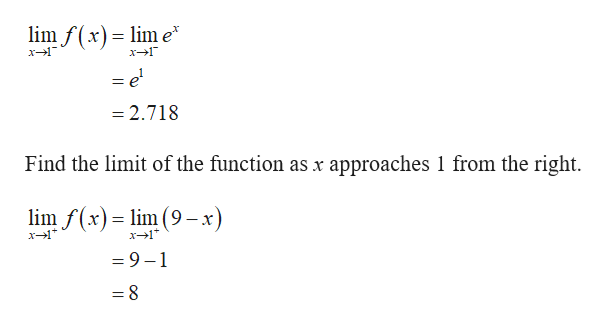# Find each x-value at which f is discontinuous and for each x-value, determine whether f is continuous from the right, or from the left, or neither.if x < 0X 2f(x)if 0 x 1exif x > 19-X(smaller value)continuous from the rightcontinuous from the leftneither(larger value)X =continuous from the rightcontinuous from the leftneither

Question
22 viewshelp_outlineImage TranscriptioncloseFind each x-value at which f is discontinuous and for each x-value, determine whether f is continuous from the right, or from the left, or neither. if x < 0 X 2 f(x) if 0 x 1 ex if x > 1 9-X (smaller value) continuous from the right continuous from the left neither (larger value) X = continuous from the right continuous from the left neither fullscreen
check_circle

Step 1

Find the limit of the function as x approaches 0 from the left.help_outlineImage Transcriptioncloselim f(x) lim x+2 x0 x0 =0 2 =2 Find the limit of the function as x approaches 0 from the rigl lim f(x)lim e x0* x0 = e 1 -1 fullscreen
Step 2

Find the limit of the function at x=0.help_outlineImage Transcriptioncloselim f(x)lme x0 x0 = e° = 1 The function is discontinuous at x 0 That is, lim f(x) lim f(x) x0 fullscreen
Step 3

Find the limit of the function as x ap...help_outlineImage Transcriptioncloselim f(x)lim e = x1 x1 = e =2.718 Find the limit of the function as x approaches 1 from the right. lim f(x)lim (9-x) x1 x1 =9-1 =8 fullscreen

### Want to see the full answer?

See Solution

#### Want to see this answer and more?

Solutions are written by subject experts who are available 24/7. Questions are typically answered within 1 hour.*

See Solution
*Response times may vary by subject and question.
Tagged in

### Other